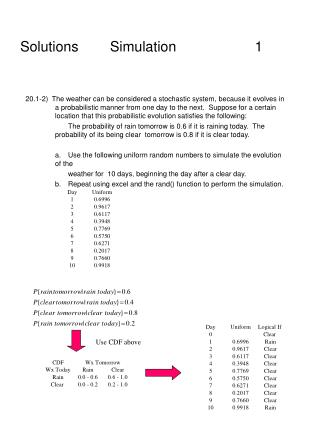DownloadDownload PresentationSolutions Simulation 1

# Solutions Simulation 1

Télécharger la présentation## Solutions Simulation 1

- - - - - - - - - - - - - - - - - - - - - - - - - - - E N D - - - - - - - - - - - - - - - - - - - - - - - - - - -
##### Presentation Transcript

1. Solutions Simulation 1 20.1-2) The weather can be considered a stochastic system, because it evolves in a probabilistic manner from one day to the next. Suppose for a certain location that this probabilistic evolution satisfies the following: The probability of rain tomorrow is 0.6 if it is raining today. The probability of its being clear tomorrow is 0.8 if it is clear today. a. Use the following uniform random numbers to simulate the evolution of the weather for 10 days, beginning the day after a clear day. b. Repeat using excel and the rand() function to perform the simulation. Use CDF above

2. Solutions Simulation 1 20.1-2) Alternate Solution Assume CDF for Rain, Clear is shown as below. CDF built on condition

3. Solutions Simulation 2 20.1-4) The William Graham Entertainment Co. will be opening a new box office where customers can come to make ticket purchases in advance for the many entertainment events being held in the area. Simulation is being used to analyze whether to have one or two clerks on duty at the box office. While simulating the beginning of a day at the box office, the first customer arrives 5 minutes after it opens and the interarrival times for the next four customers are 3 minutes, 1 minute, and 4 minutes. The service times are 8 minutes, 6 minutes, 2 minutes, 4 minutes, and 7 minutes. a. Plot the no. of customers at the box office over time. b. Estimate L, Lq, W, and Wq for this queueing system. c. Repeat for two clerks.

4. Solutions Simulation 3 20.1-4) solution a.) L = 1.25, Lq = 0.41,

5. 20.1-4) solution

6. Solutions Simulation 5 20.3-1) Use the mixed congruential method to generate the following sequence of random numbers. a. 10 one-digit integer numbers such that Xn+1 = (Xn + 3) modulo 10 and Xo = 2. b. 8 integers (0-7) such that Xn+1 = (5Xn + 1) modulo 8 and Xo = 1.

7. Solutions Simulation 6 20.3-1) Use the mixed congruential method to generate the following sequence of random numbers. c. 5 two-digit integer numbers such that Xn+1 = (61Xn + 27) modulo 100 and Xo=10.

8. 1.0 0.8 .06 0.4 0.2 7 9 11 13 Solutions Simulation 7 20.4-11) Consider the following CDF. U1 = .2655 X1 = 9.2 U2 = .3472 X2 = 9.5 U3 = .0248 X3 = 7.0 U4 = .9205 X4 = 12.2 U5 = .6130 X5 = 10.5